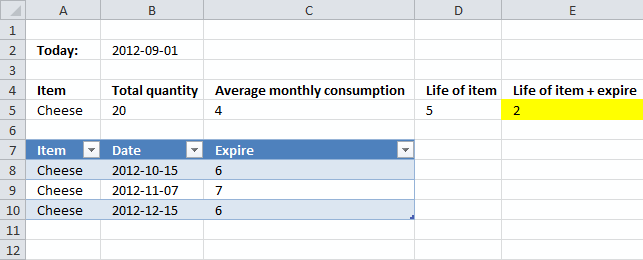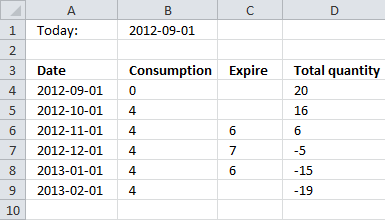Author: Oscar Cronquist Article last updated on October 12, 2012

Haroun asks:

The problem is with regards to analysing the remaining life of my inventory based on the quantity available and the monthly consumption. Its as follows :

Assuming today's date is 1st of September. I have 20 packets of cheese and the average monthly consumption is 4 packets. Ideally if the shelf life of those 20 packets is 6 months or more, then I can safely say that the total quantity will last for 5 months (20 divided by 4).However, with a slight twist in the data, assume that out of those 20 packets, 6 packets expire on 15 Oct, 7 packets expire on 10 Nov, and the remaining 6 on 15 December. Now I know that the total inventory is not gonna last for 5 months due to various expiry dates. If I calculate this manually, I come to know that the quantity will last for 3 and a half months.

How can i put all this in a formula to get the right answer ?

Please advise.
Thanks
Haroun

Answer:Array formula in cell E5:

=(YEAR((MAX(IF((((ROW(\$1:\$99)-1)*\$C\$5)+SUMIF(Table1[Date], "<="&DATE(YEAR(B2), MONTH(B2)+ROW(1:99)-1, DAY(B2)), Table1[Expire]))<B5, DATE(YEAR(B2), MONTH(B2)+ROW(1:99)-1, DAY(B2)), ""))))-YEAR(B2))*12+MONTH(MAX(IF((((ROW(\$1:\$99)-1)*\$C\$5)+SUMIF(Table1[Date], "<="&DATE(YEAR(B2), MONTH(B2)+ROW(1:99)-1, DAY(B2)), Table1[Expire]))<B5, DATE(YEAR(B2), MONTH(B2)+ROW(1:99)-1, DAY(B2)), "")))-MONTH(B2)

This formula returns months, rounded down. It can calculate up to 99 months. So how did the formula come up with the value 2? Take a look at this table.Today is 2012-09-01, a month later (2012-10-01) 4 items are consumed and 0 items have expired, total quantity 16.

2012-11-01 a total of 8 items are consumed and 6 items have expired, total quantity 6.

2012-12-01 a total of 12 items are consumed and 13 items have expired, total quantity -5.

All the inventory items are consumed/expired somewhere between 2012-11-01 and 2012-12-01.

2012-11-01 - 2012-09-01 = 2 months

### Download excel *.xlsx file

Inventory consumption.xlsx